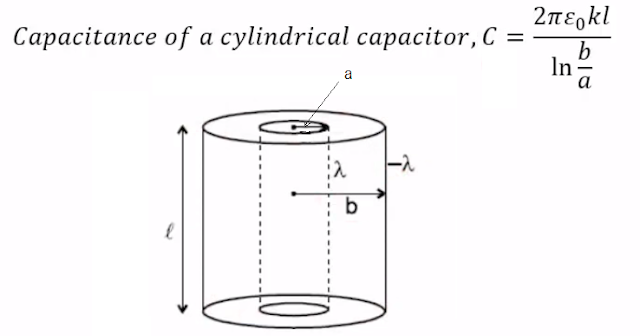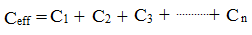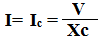## Capacitance Formula

There are much Capacitance Formulas. In this article, you will find all the basic capacitance formulas and equations together. These all equations and formulas are very important. Before connecting capacitors in any electrical circuits, the calculation must be needed. There are many uses of a capacitor like in filter circuit, power factor improvement circuit etc. For all applications of capacitor needs the formula and equations.

Capacitance: Capacitance is the property of a capacitor by virtue of which the capacitor can store electrical energy in form of electrical charge.

The Unit of Capacitance is Farrad which is denoted by 'F'C = coulomb
V = volt

One(1) Coulomb of charge stored per One Volt applied voltage.

When we apply an electrical voltage to the capacitor then the capacitor stores the electrical energy in form of electrical charge.

So we can write,Where
C= capacitance
Q= charge
V= Voltage or potential difference

Based on the physical characteristics of the capacitor, the capacitance formula is,Here,
k= relative permittivity
ε= permittivity of free space
A = surface area of plates
d=distance between platesThe energy storing formula of the capacitor is,### Capacitors connected in Series:

When a no. of capacitors are connected in series then the formula of capacitance will be,### Capacitors connected in Parallel:

When a no. of capacitors are connected in parallel then the formula of capacitance will be,### Capacitor Reactance Formula:

The property of a capacitor by virtue of which the capacitor can oppose the flow of alternating current(AC) is called capacitance reactance.
The capacitive reactance is denoted by XcHere,
C= Capacitance
f= frequency
The unit of capacitive reactance is ohm

If an AC circuit has the only capacitor the equation of current will be,Capacitance Formula | All Formulas and Equations ExplainReviewed by Author on January 13, 2019 Rating: 5